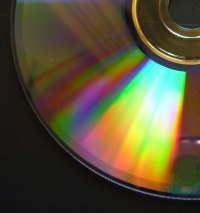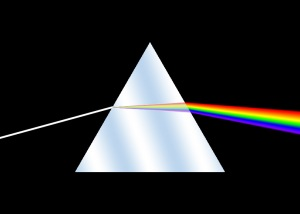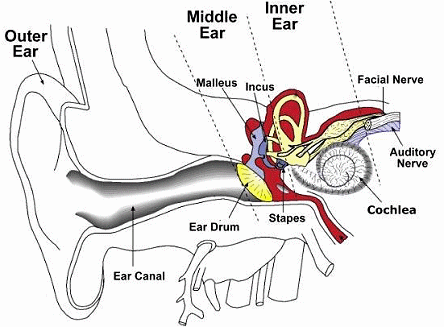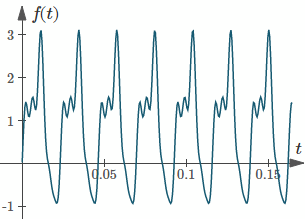Search IntMath
Close

450+ Math Lessons written by Math Professors and Teachers

5 Million+ Students Helped Each Year

1200+ Articles Written by Math Educators and Enthusiasts

Simplifying and Teaching Math for Over 23 Years

# 7. Application - The Fast Fourier Transform

## 1. Digital AudioCD-ROM

Pulse code modulation (PCM) is the most common type of digital audio recording, used to make compact disks and WAV files.

In PCM recording hardware, a microphone converts sound waves into a varying voltage. Then an analog-to-digital converter samples the voltage at regular intervals of time. For example, in a compact disc audio recording, there are 44100 samples taken every second.

The data that results from a PCM recording is a function of time. How does this work?

Imagine that you were very small and could fit into your friend's ear drum. Suppose also that you could see things in very slow motion and that you could record the position of the ear drum once every 44100th of a second. Your eyes are so good that you can notice 65536 distinct positions of the ear drum's surface as it moves back and forth in response to incoming sound waves.

If your friend is listening to the sound of a flute, and you write down the positions of the ear drum that you notice, then you would have a digital PCM recording - a series of numbers.

If you could later make your own ear drum move back and forth in accordance with the thousands of numbers you had written down, you would hear the flute exactly as it originally sounded. We have gone from:

rich sound with fundamentals and harmonics
→ numbers
→ rich sound with fundamentals and harmonics

To be able to convert from the series of numbers to sound, we need to apply the Fourier Transform.

## 2. Frequency Information as a Function of Time

### Prisms

One analogy for the type of thing a Fourier Transform does is a prism which splits white light into a spectrum of colors.

White light consists of all visible frequencies (red, orange, yellow, green, blue, indigo and violet) mixed together (much like the information on a CD has sounds of all frequencies mixed together) and the prism breaks them apart so we can see the separate frequencies (much like the CD player splits apart the sound frequencies so they can be amplified and sent to the speakers).White light is split into individual frequencies by a prism

### Cochlea

In our inner ears, the cochlea enables us to hear subtle differences in the sounds coming to our ears. The cochlea consists of a spiral of tissue filled with liquid and thousands of tiny hairs which gradually get smaller from the outside of the spiral to the inside. Each hair is connected to a nerve which feeds into the auditory nerve bundle going to the brain. The longer hairs resonate with lower frequency sounds, and the shorter hairs with higher frequencies. Thus the cochlea serves to transform the air pressure signal experienced by the ear drum into frequency information which can be interpreted by the brain as tonality and texture.The cochlea transforms sound waves into electrical signals.

The Fourier Transform is a mathematical technique for doing a similar thing - resolving any time-domain function into a frequency spectrum. The Fast Fourier Transform is a method for doing this process very efficiently.

## 3. The Fourier Transform

As we saw earlier in this chapter, the Fourier Transform is based on the discovery that it is possible to take any periodic function of time f(t) and resolve it into an equivalent infinite summation of sine waves and cosine waves with frequencies that start at 0 and increase in integer multiples of a base frequency f_0= 1/T, where T is the period of f(t). The resulting infinite series is called the Fourier Series:

f(t) =a_0/2+sum_(n=1)^oo(a_n\ cos nt {:+b_nsin nt)

The job of a Fourier Transform is to figure out all the an and bn values to produce a Fourier Series, given the base frequency and the function f(t).

In our CD example, which has a sampling rate of 44100 samples/second, if the length of our recording is 1024 samples, then the amount of time represented by the recording is 1024/44100=0.02322 seconds, so the base frequency f0 will be

1/T=44100/1024=43.066\ "Hz".

If you process these 1024 samples with the FFT (Fast Fourier Transform), the output will be the sine and cosine coefficients an and bn for the frequencies

43. 066\ "Hz",

2 × 43. 066 = 86. 132\ "Hz",

3 × 43. 066 = 129. 20\ "Hz", etc.

### Example

Let's say that we use the FFT to process a series of numbers on a CD, into a sound.

It may give us something like a_0= 1.6, a_n=((-1)^n)/n and b_n=1/(2n-1.

For the frequencies 43.066\ "Hz", 86.123\ "Hz" and 129.20\ "Hz", we use T=(2pi)/b, and we have:

b=(44100(2pi))/1024=270.59

So the Fourier Series would be:

f(t) =1.6/2+sum_(n=1)^5(((-1)^n)/ncos 270.59nt {:+1/(2n-1)sin 270.59nt)

= 0.8 − 1.0 cos 270.59t + sin 270.59t +\ 0.5 cos 541.18t +\ 0.33333 sin 541.18t  −\ 0.33333cos 811.77t +\ 0.2sin 811.77t +\ 0.25cos 1082.4t  +\ 0.14286sin 1082.4t  −\ 0.2 cos 1353.0t  + 0.11111 sin 1353.0tGraph of the first few terms of f(t) (for n=1 to n=5).

We have reconstructed a sound wave from the digital data fed from the CD into the sound system of the CD player.

## Problem SolverThis tool combines the power of mathematical computation engine that excels at solving mathematical formulas with the power of GPT large language models to parse and generate natural language. This creates math problem solver thats more accurate than ChatGPT, more flexible than a calculator, and faster answers than a human tutor. Learn More.